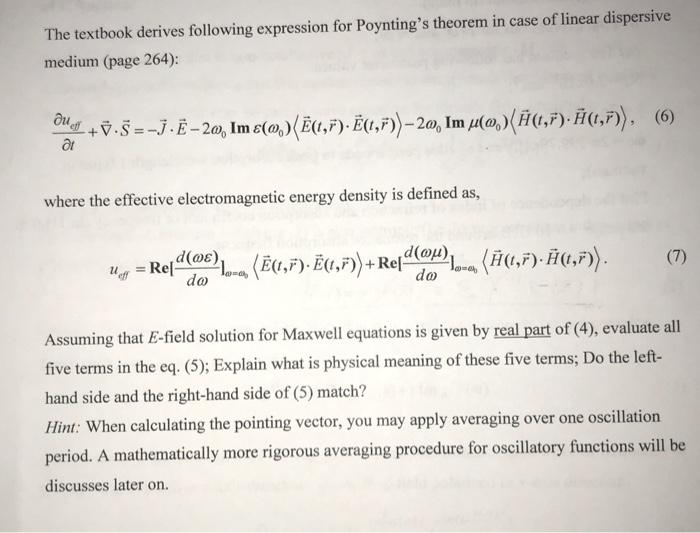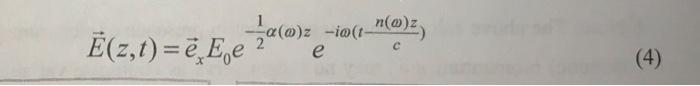# (Solved): The question is supposed to read "evaluate all five terms in the equation (6)" The textbook derives ...The question is supposed to read "evaluate all five terms in the equation (6)"
The textbook derives following expression for Poynting's theorem in case of linear dispersive medium (page 264): $\frac{\partial u_{e \ddot{f}}}{\partial t}+\vec{\nabla} \cdot \vec{S}=-\vec{J} \cdot \vec{E}-2 \omega_{0} \operatorname{Im} \varepsilon\left(\omega_{0}\right)\langle\vec{E}(t, \vec{r}) \cdot \vec{E}(t, \vec{r})\rangle-2 \omega_{0} \operatorname{Im} \mu\left(\omega_{0}\right)\langle\vec{H}(t, \vec{r}) \cdot \vec{H}(t, \vec{r})\rangle,$ where the effective electromagnetic energy density is defined as, $u_{e f f}=\operatorname{Re}\left[\frac{d(\omega \varepsilon)}{d \omega}\right]_{\omega=\omega_{0}}\langle\vec{E}(t, \vec{r}) \cdot \vec{E}(t, \vec{r})\rangle+\operatorname{Re}\left[\frac{d(\omega \mu)}{d \omega}\right]_{\omega=\omega_{b}}\langle\vec{H}(t, \vec{r}) \cdot \vec{H}(t, \vec{r})\rangle .$ Assuming that $$E$$-field solution for Maxwell equations is given by real part of (4), evaluate all five terms in the eq. (5); Explain what is physical meaning of these five terms; Do the lefthand side and the right-hand side of (5) match? Hint: When calculating the pointing vector, you may apply averaging over one oscillation period. A mathematically more rigorous averaging procedure for oscillatory functions will be discusses later on. $$\vec{E}(z, t)=\vec{e}_{x} E_{0} e^{-\frac{1}{2} \alpha(\omega) z} e^{-i \omega\left(t \frac{n(\omega) z}{c}\right)}$$

We have an Answer from Expert